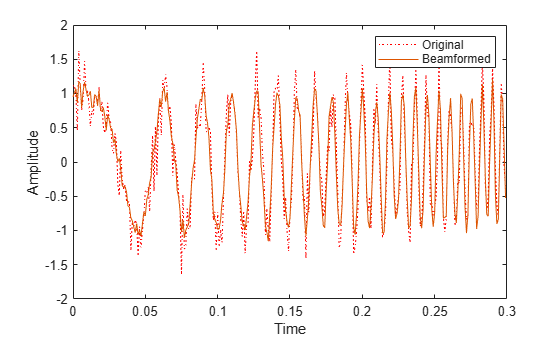Documentation

# step

System object: phased.SubbandPhaseShiftBeamformer
Package: phased

Beamforming using subband phase shifting

## Syntax

```Y = step(H,X) Y = step(H,X,ANG) [Y,W] = step(___) [Y,FREQ] = step(___) [Y,W,FREQ] = step(___) ```

## Description

### Note

Starting in R2016b, instead of using the `step` method to perform the operation defined by the System object™, you can call the object with arguments, as if it were a function. For example, ```y = step(obj,x)``` and `y = obj(x)` perform equivalent operations.

`Y = step(H,X)` performs subband phase shift beamforming on the input, `X`, and returns the beamformed output in `Y`.

`Y = step(H,X,ANG)` uses `ANG` as the beamforming direction. This syntax is available when you set the `DirectionSource` property to `'Input port'`.

```[Y,W] = step(___)``` returns the beamforming weights, `W`. This syntax is available when you set the `WeightsOutputPort` property to `true`.

```[Y,FREQ] = step(___)``` returns the center frequencies of subbands, `FREQ`. This syntax is available when you set the `SubbandsOutputPort` property to `true`.

```[Y,W,FREQ] = step(___)``` returns beamforming weights and center frequencies of subbands. This syntax is available when you set the `WeightsOutputPort` property to `true` and set the `SubbandsOutputPort` property to `true`.

### Note

The object performs an initialization the first time the object is executed. This initialization locks nontunable properties (MATLAB) and input specifications, such as dimensions, complexity, and data type of the input data. If you change a nontunable property or an input specification, the System object issues an error. To change nontunable properties or inputs, you must first call the `release` method to unlock the object.

## Input Arguments

 `H` Beamformer object. `X` Input signal, specified as an M-by-N matrix. If the sensor array contains subarrays, N is the number of subarrays; otherwise, N is the number of elements. This argument can be specified as single or double precision.The size of the first dimension of the input matrix can vary to simulate a changing signal length. A size change can occur, for example, in the case of a pulse waveform with variable pulse repetition frequency. `ANG` Beamforming directions, specified as a two-row matrix. Each column has the form [AzimuthAngle; ElevationAngle], in degrees. Each azimuth angle must be between –180 and 180 degrees, and each elevation angle must be between –90 and 90 degrees. This argument can be specified as single or double precision.

## Output Arguments

 `Y` Beamformed output. `Y` is an M-by-L matrix, where M is the number of rows of `X` and L is the number of beamforming directions. This argument can be returned as single or double precision. `W` Beamforming weights. `W` has dimensions N-by-K-by-L. K is the number of subbands in the `NumSubbands` property. L is the number of beamforming directions. If the sensor array contains subarrays, N is the number of subarrays; otherwise, N is the number of elements. Each column of `W` specifies the narrowband beamforming weights used in the corresponding subband for the corresponding direction. This argument can be returned as single or double precision. `FREQ` Center frequencies of subbands. `FREQ` is a column vector of length K, where K is the number of subbands in the `NumSubbands` property. This argument can be returned as single or double precision.

## Examples

expand all

Apply subband phase-shift beamforming to an 11-element underwater ULA. The incident angle of a wideband signal is 10° in azimuth and 30° in elevation. The carrier frequency is 2 kHz.

Create the ULA.

```antenna = phased.ULA('NumElements',11,'ElementSpacing',0.3); antenna.Element.FrequencyRange = [20 20000];```

Create a chirp signal with noise.

```fs = 1e3; carrierFreq = 2e3; t = (0:1/fs:2)'; x = chirp(t,0,2,fs); c = 1500; collector = phased.WidebandCollector('Sensor',antenna, ... 'PropagationSpeed',c,'SampleRate',fs,... 'ModulatedInput',true,'CarrierFrequency',carrierFreq); incidentAngle = [10;30]; x = collector(x,incidentAngle); noise = 0.3*(randn(size(x)) + 1j*randn(size(x))); rx = x + noise;```

Beamform in the direction of the incident angle.

```beamformer = phased.SubbandPhaseShiftBeamformer('SensorArray',antenna, ... 'Direction',incidentAngle,'OperatingFrequency',carrierFreq, ... 'PropagationSpeed',c,'SampleRate',fs,'SubbandsOutputPort',true, ... 'WeightsOutputPort',true); [y,w,subbandfreq] = beamformer(rx);```

Plot the real part of the original and beamformed signals.

```plot(t(1:300),real(rx(1:300,6)),'r:',t(1:300),real(y(1:300))) xlabel('Time') ylabel('Amplitude') legend('Original','Beamformed')```Plot the response pattern for five frequency bands.

```pattern(antenna,subbandfreq(1:5).',[-180:180],0,'PropagationSpeed',c, ... 'CoordinateSystem','rectangular','Weights',w(:,1:5)) legend('location','SouthEast')```## Algorithms

The subband phase shift beamformer separates the signal into several subbands and applies narrowband phase shift beamforming to the signal in each subband. The beamformed signals in all the subbands are regrouped to form the output signal.

For further details, see .

## References

 Van Trees, H. Optimum Array Processing. New York: Wiley-Interscience, 2002.